Aids to Understanding

Fields in physics

In physics when we speak of a field, for example a gravitational field or magnetic field we are talking about a region of space in which a body experiences a force. A mass will experience an attractive force in a gravitational field and an iron based metal will experience a force in a magnetic field. The source of a gravitational field is a mass and the source of a magnetic field is either a permanent magnet or a current carrying conductor. To help us visualize electric fields we use arrows. The direction of the arrow is the direction a relevant body will experience a force. A gravitational field due to the Earth is shown below. The force experienced by a mass is in the direction of the field.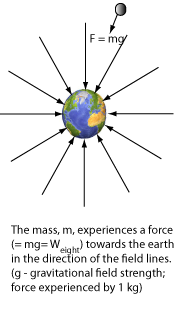Energy matter

A free charge will experience a force and, if in a vaccum, will therefore accelerate. The energy change is electrical energy to moving energy. However if the electric field is applied to a conductor, the free charges do not accelerate due to the natural resistance of the conductor (ie the extent free charges are available in the conductor and the extent they collide with the stationary atomic nuclei). In this case the energy change is electric energy to heat energy.

Electric Current

Electric current, I, is defined as Q/t where Q is measured in Coulombs and t is measured in seconds. So if a current of 6 A is flowing in a wire then 6 C of charge drifts past each point every second.
1 C = 1.6 X 1018 charges. Most of you should know that the higher the resistance the lower the current but it should be clear that the current is the same at all points in a series circuit, including the wires and the resistors.

Working against the gravitational field

When a force is doing work on a body it is transferring energy to that body. For example an unbalanced force of 6N pulling a toy car for 2 m, will have transfer 12 J of energy and the car will have acquired 12 J of kinetic energy (see 1.3). If the force was balanced then 12 J of heat energy will be produced and there is no change in kinetic energy. However, if a balanced force works on a body and no heat is produced, then the energy transferred is stored in the body as potential energy. This is the situation when a force of 9.8 N raises a mass of weight 9.8 N a height of 1 m. The balanced lifting force does 9.8 J of work against the gravitational field and this is stored in the weight as gravitational potential energy.

Potential Energy and Potential Difference

You will have noticed that in this unit energy matters have arisen frequently, but now we are suddenly speaking of potential difference that have units of Volts (not Joules). And when we numerically label an electric field arrangement, the numbers have units of V. The diagram on the right is labelled 100 V to 260 V. Why not simply label it 100 J to 260 J, you may ask? Well the point is when we label something it must be the same for everything relevant to it. For example a height of 6 m does not depend on what is sitting at that height and all bodies sitting there are a height of 6 m, but the potential energy of a body at that height also depends on the mass of the body. In an electric field context the Energy per Coulomb (potential) is the same for all charges at a given point in the field (ie our dotted lines). For example suppose a 2 C and a 5 C charge are sitting at a potential of 15 V, what is the potential energy of each charge? The answers are 30 J and 75 J. To get our answer we used Ep = QV = Ew. If both charges sat at zero potential initially then 30 J and 75 J of Work had to be done to place the charges at point 15 V.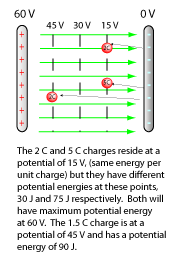It should be clear that work done moving a charge between two points equals change in potential energy of the charge and is proportional to the potential difference between the points. You should see how this is similar to the gravitational context.

Finding the speed of a particle

The speed of a charged particle is worked out by invoking the principle of conservation of energy. When a charge falls through a pd it loses potential energy and gains kinetic energy. If the particle is initially at rest we can say QV = 1/2mV2.
Example an electron of mass 9.1 x 10-31 kg and charge 1.6 x 10-19 C falls through a pd of 1000.0V.
i) Calculate the work done by the field. Answer, QV = 1.6 x 10-19. This is equal to increase in Ek.
ii) Calculate the electron's velocity.
Answer, 1.6 x 10-19 = 1/2mv2, V = 5.3 x 106ms-1
Note: the speed can never exceed 3.0 x 108 so if you find you have an answer too big, you have probably forgotten to take the square root.

Electro Motive Force and Internal resistance

EMF is defined in terms of joules per coulomb and not in Newtons. The usefulness of defining a cell's action in terms of Forces is that it reminds us of the forces inside cell due to the cell's chemical actions. These forces displace charges through electric field and give them electric potential energy. When these forces also work against the cell's internal resistance then heat energy is also produced.
The energy change per coloumb is therefore Chemical to electric energy and heat, from this is follows that the total energy supplied to each coulomb, E, is given by
E = V + Lost volts.
Convince yourself of the validity of this expression.

Straight line Mathematics and investigative ideas

The equation of a straight line is y = mx + c. This means the y value will change if the x value changes. The m and c are constant and represent the gradient of the line and c is where the line cuts the y-axis at x = 0. Now the expression for a real cell is given by
E = Vtpd + Ir or
Vtpd = -rI + E. This means if we change I the Vtpd will change, -r will be the gradient of the line and the vertical axis intercept is the EMF.EMF and Internal Resistance problem

The cell in the circuit below has an EMF of 3.0 V. From circuit (a) find the value of the load resistance R and internal resistance r. In circuit (b) and (c) the circuits are modified with a resistor identical to resistor R in circuit (a) Find the readings on the ammeters and voltmeters in both cases.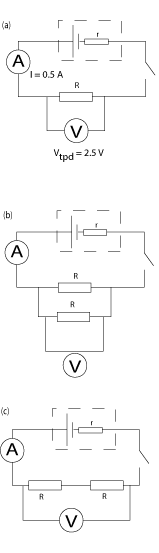Resistors in Parallel and Series

Work out the value of resistor is equivalent or equal to the resistors in each network below and pro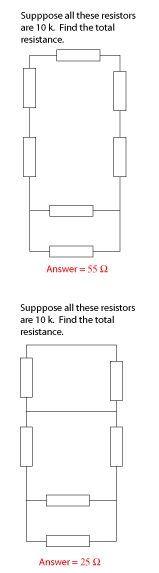The voltage divider

If we have two resistors in series with a source of EMF, then
i) the potential drop across both resistors add up to the EMF.
ii)the current is each resistor is the same.
Use these facts to show that the ratio of potential drops across each resistor equals the ratio of the resistances. ie
V1/V2 = R1/R2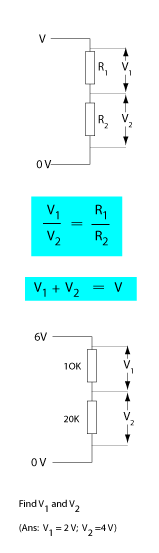Description in Physics

Suppose you were given a resistor of resistance 200 W, for example, and were asked to explain what 200 W meant. To answer this properly you must quantify in terms of a unitary quantity, in this case our unitary quantity is Amps. You would write R = V/I and say 200 W means 200 V A-1. In other words if we wish 1 A to flow in our 200 W resistor we need to apply 200 V across its terminals. If we have a 400 W resistor then we need 400 V before 1.0 A will flow in it. When you are asked to write units after a quantity, you are writing the meaning of the quantity that you have calculated.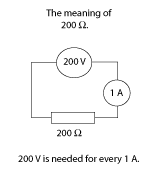## 1) Electric fields

An electric field is a a region of space in which a charged particle experiences a force. The source of an electric field is a positively or negatively charged body and the imaginary electric field lines that either emanate from the source or point to the source is defined as the direction of the force that a positively charged Particle will experience in this Field, ie FPF. The closeness of the field lines indicate the magnitude of this force. The diagram below helps illustrate this.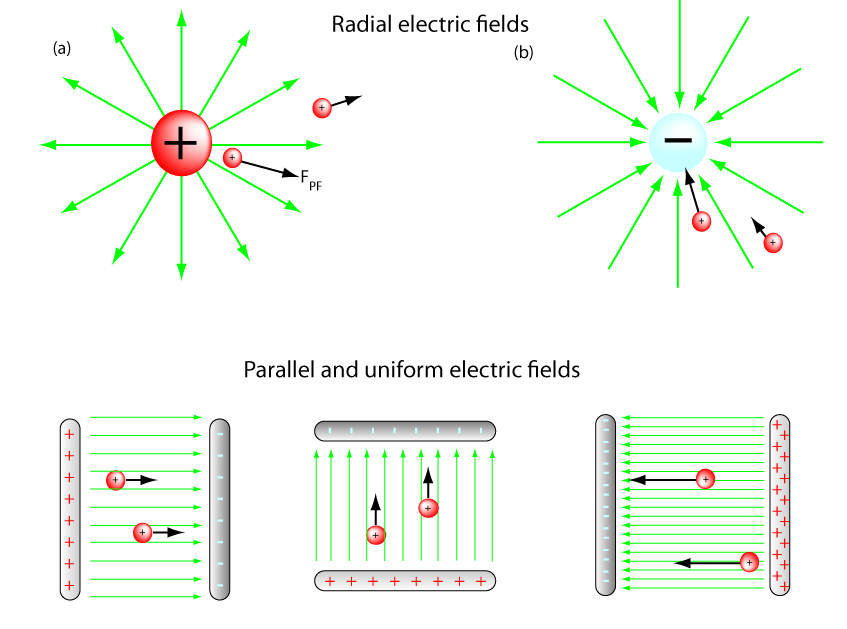The green arrows show the electric field and the black vectors show the direction of the force on the particle. Note how the force on a positive charge is always in the direction of the field and its magnitude will get greater as the lines of force get closer. This follows for both radial and parallel fields. You should notice that for a uniform parallel field the field lines are at the same distant apart therefore the force on a charged particle is independent of where the charge is. You are invited to draw the same diagrams with a negative charge,

## 2) Electric fields and current

The diagram below shows that electric fields cause free electric charges to flow in wires (conductors). The charges are always in motion (called thermal motion due to the temperature of the wire) but do not flow in a particular direction unless an electric field is present. The source of the field in this context is normally a cell.Resistance and localising the field Suppose we have a long conductor made from three parts all connected in series. Part (i and iii) have very low resistances (0.1 W) part (ii) has a very high resistance (100 W). One important assumption we must make is that the current is the same at all points. This is necessary or we would be losing or gaining charge somewhere inside this material.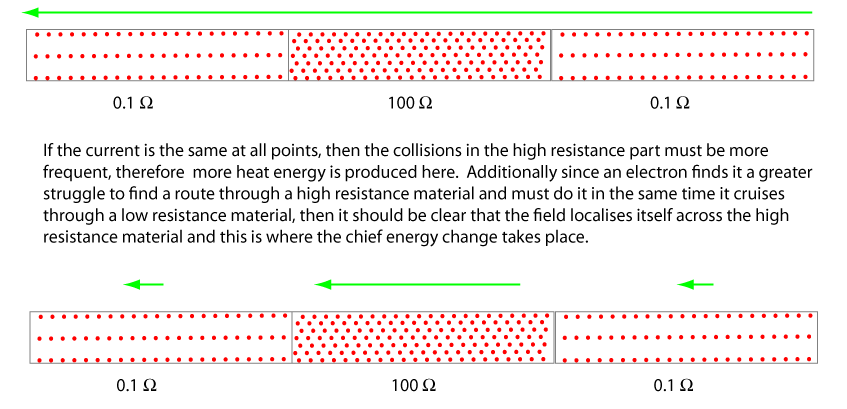It may have occurred to you that the diagram above is simply a resistor between two wires. We will return to this argument when discussing voltage rules (see 11) below) for series and parallel circuits.

## 3) Electric fields and energy

This unit is about electrical energy and its transfer from cells to circuit components like bulbs and heaters. It is important therefore to appreciate why charges in electric fields have energy. First we consider the simplest case where the electric field is in a vacuum. When we study electric circuits we will see how the arguments are similar when the fields, produced by cells, are in circuit elements and wires. If a balanced force displaces a positive charge against the field lines then the force is doing work (transferring energy) on the charge and the charge acquires electric potential energy. If the positive charge is released and allowed to follow the field lines then the charge will experience an unbalanced force and acquire kinetic energy. The energy change is electric potential to kinetic energy. This is identical to the argument in the left hand side column for masses in gravitational fields.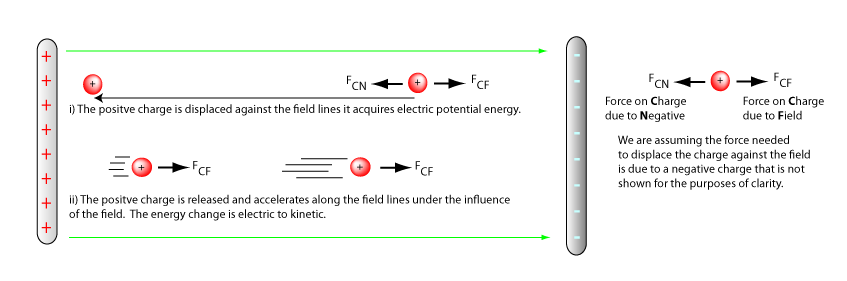The force displacing the positive charge has done work, W, and energy has been stored in it as potential energy. When the charge is released the unbalanced force due to the field does work and it acquires kinetic energy as it travels along the field lines.

## 4) Potential difference

When calculating the gravitational potential energy (work done) acquired by a body we use the expression EW = (mg).h. It is clear that the higher the height then the greater the work done. In this unit we wish to calculate the work done on a charge as it moves between two points n an electric field. We find it depends on the magnitude of the charge Q and the potential difference the charge moves through. Potential difference to electricity is what height is to mechanics. For example the greater the potential difference a charge moves through the greater the work done (energy transferred). Potential difference can be written as DV = Vf - Vi (V- volts, f-final, i - initial) however often the intial potential is zero so we just write, V.
Example: Suppose a charge resides at a point where the potential is 140 V and a force moves it to a point where its potential is 240 V. What potential difference did the charge move through?
DV = Vf - Vi = (240 - 140) = 100 V## 5) The meaning of voltage

If the potential difference between two points is 1 V, then we say 1 J of energy is needed to move 1 C of charge between those points. From this definition you should see that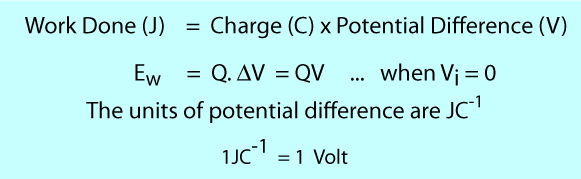Example Suppose an electron of charge magnitude of 1.6 x 10-19 C moves through a potential difference (voltage) of 1 V. If the electron is moving along the field lines, how much work was done on the electron? The answer is 1.6 x 10-19J and we call this 1 eV or 1 electronvolt.

## 6) Potential difference and Energy calculations

The two contexts we can apply our potential energy and potential difference relationships, are charges accelerating through through vacuums and charges drifting through circuits. In both cases the charges are moving under the influence of electric fields but the energy changes are different.
i) Electric fields in vacuums
Suppose an alpha particle resides at 140 V in the electric field below. If the particle is allowed to fall with the field, then..
(i) Calculate is potential energy as it passes through the 80 V and 40 V points
(ii) Calculate the speed of the particle at these points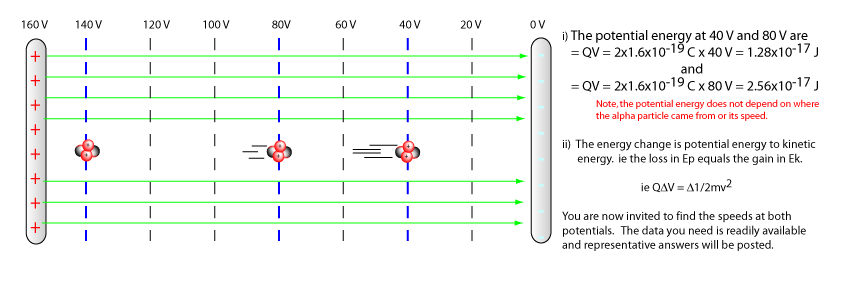ii) Electric fields in circuits
Suppose a 1.0 kW resistor has a current of 2.0 A flowing in it and a potential difference (voltage) across it of 6.0 V.
(i) Calculate the heat energy produced each time 1 C of negative charge flows across this pd.
(ii) Calculate the heat energy produced per second.
Answer (i) The energy change is electric potential energy to heat energy (the charges do not change speed as they traverse an electric field in a conductor). The heat energy produced is still given by QV = 6.0 J.
Answer (ii) If 1 C passing through the 6.0 V pd produces 6 J of heat energy then 2 Coulombs passing through per second produce 12.0 Joules of heat energy every second, ie 12.0 Js-1. This should be familiar to you as P = I.V. You should now begin to see the important energy distinctions between charges flowing through vacuums and charges flowing through conductors. You should also be able to see the principle of conservation of energy in action in both cases.

## 7) E M F - Electromotive Force

Any device, such as a dry cell, solar cell or thermocouple that can change one form of energy into electric potential energy is called a seat or source of EMF. In this unit we chiefly concern ourselves with dry cells. Suppose you pick up a small 1.5 V battery, what does the 1.5 V mean? It means that the battery will provide 1.5 J of energy to each Coulomb of charge that passes through it ie from 0 V to 1.5 V. We say the battery has an EMF OF 1.5 V. To increase the potential of a charge then the cell must do work on the charge against the field and this work is done by chemical reactions.

## 8) Internal resistance of a cell

When a cell of EMF 1.5 V is delivering energy to an external circuit we find the work done on the charge as it travels from the lower to higher potential in the cell is less than we expect. In other words we get less than 1.5 J for every Coulomb, even though the cell still does 1.5 J of work on each Coulomb. To explain this we introduce a property of a cell called internal resistance. To explain how internal resistance reduces the potential difference between the cell's terminals, a force argument can be used. It is important to remind yourself that charges never accelerate in cells or conductors, ie the forces acting on then are always balanced.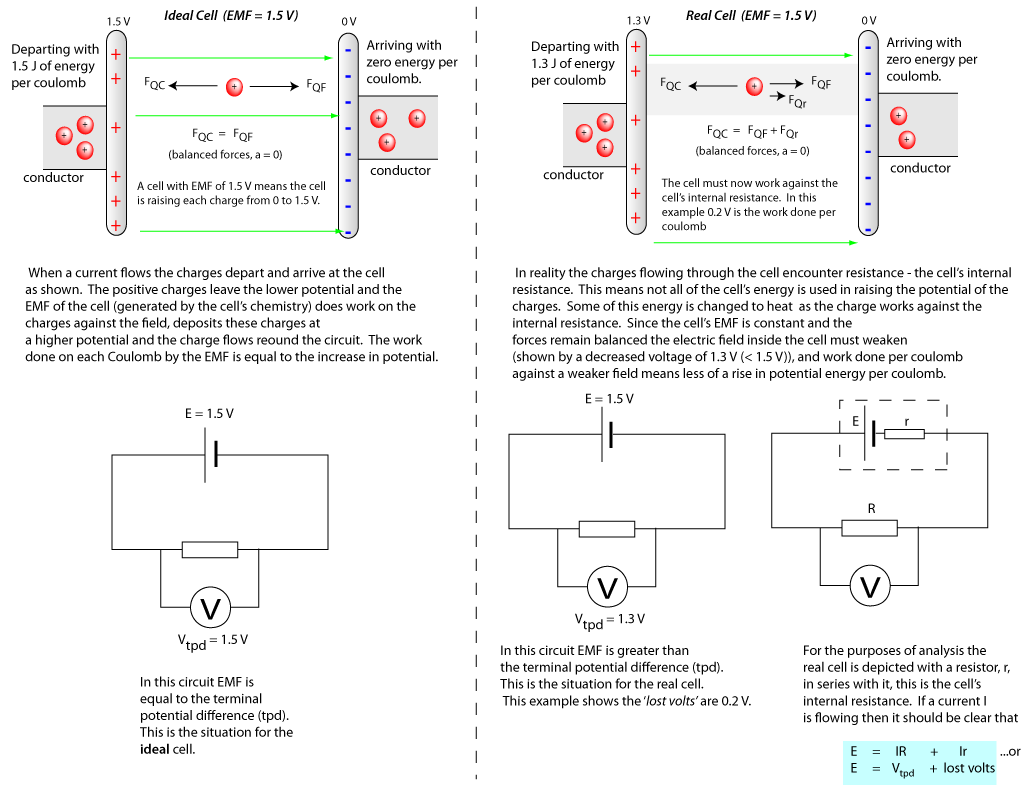## 9) Finding the EMF and internal resistance of a cell

The following circuits can be used to find the EMF and internal resistance of a cell. In method 1 the variable resistor is changed and the Vtpd is measured for a range of currents. The results are plotted and from the graph we find the internal resistance and EMF of the cell. In method 2 we change the resistance and record the current. You are invited to find the EMF and internal resistance of this cell.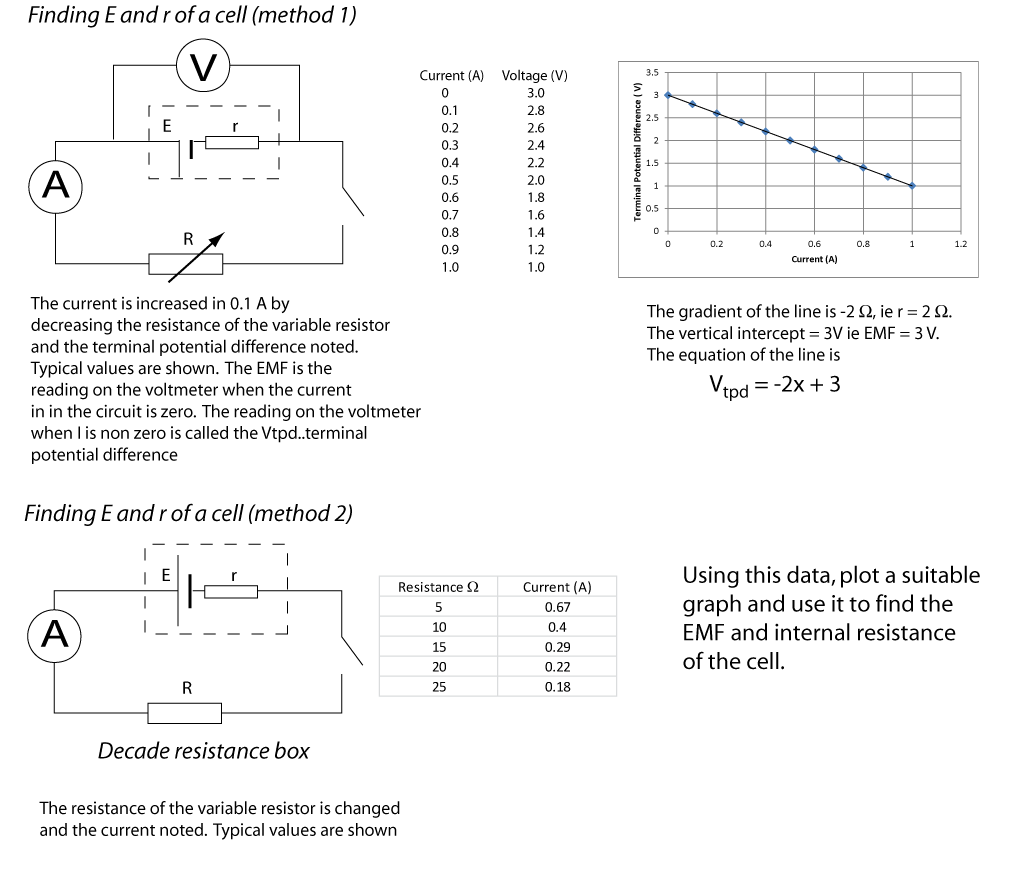## 10) Open circuits and EMF

It should be clear from earlier discussions that the two constants of a cell are its internal resistance and EMF. As the current decreases the lost volts decrease and utimately become zero when the current is zero and the current is only zero when the circuit is open. If the lost volts are zero then the potential difference between the terminals of the cell is equal to the EMF of the cell. Therefore to find the EMF of a cell connect a voltmeter across it and since I = 0 A, the reading will be the EMF. This is entirely consistent with the tables and graphs for Method 1, above.## 11) Conservation of energy and electric circuits

The principle of the conservation of energy and the conservation of charge govern and account for all electric circuit phenomenon. Suppose we have a source of EMF and it raises each Coulomb of charge to a potential of E volts, if however, that Coulomb is now provided with a alternative route to the cell's lower potential through a series of resistors (say) then two things must hold;
(i) The sum of the work done on each coulomb by the electric fields across each resistor is equal to the total energy supplied to each coulomb by the cell's EMF. (Conservation of Energy)
(ii) The current is the same at all points including the cell and the resistors.(Conservation of charge)
We are assuming the cell is an ideal cell with no internal resistance and the connecting wires also have no resistance, or a resistance so much lower than the circuit resistances thaty it can be ignored. You may modify this argument for a non-ideal cell and wires with a non-zero resistance, if you wish. These points are illustrated below.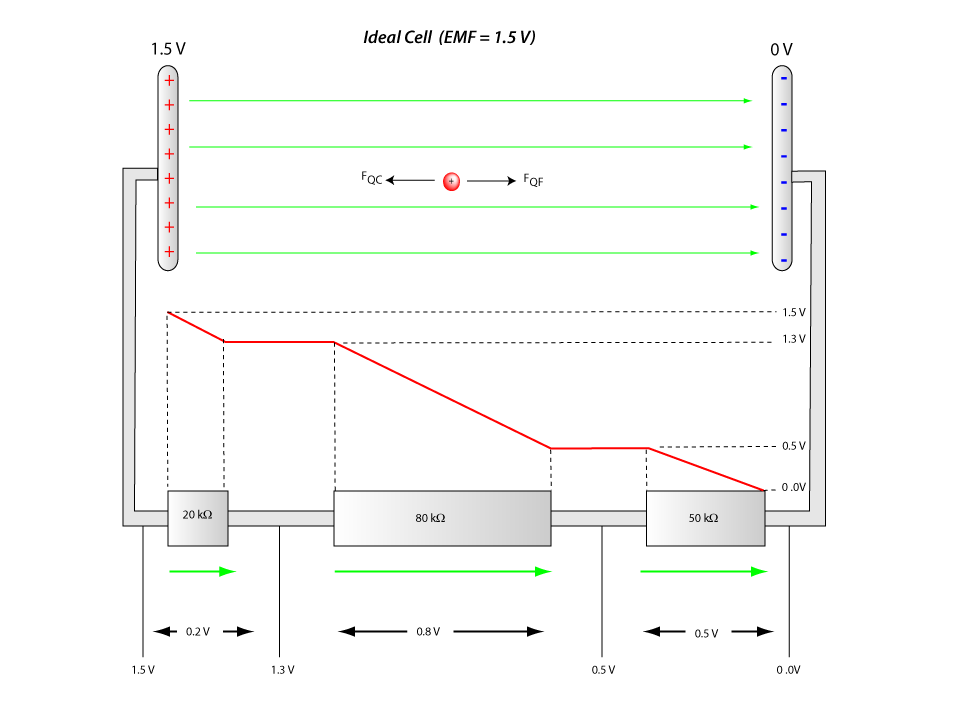The red graph shows the potential (energy/coulomb) 'drop' across each resistor. The electric field (shown in green) does work on each charge as it travels through each resistance and maintain the constant current. The charge loses electrical energy as it falls to lower and lowere potentials. The energy change is electrical energy to heat energy. The resistors are drawn at different lengths to illustrate the fact that higher value resistors create larger potential drops and therefore have larger fields across them (See 2) above). It should be clear that the the conservation of energy was used to put the diagram together and sum of the potential drops is equal to the EMF of the cell.

## 12) Resistors in series

The arguments above quickly lead to the expression for the sum of resistors in series.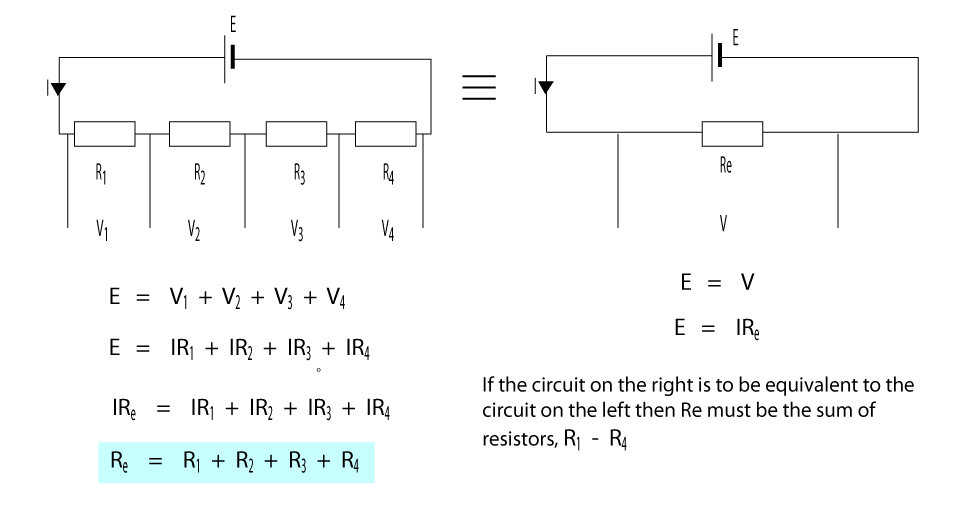If we have a number of resistors in series and we wish to replace it with one equivalent resistor that will allow the same current and potential drop then this resistor will be the the sum of the resistors it replaces. The conservation of charge leads to the constancy of the current in each resistor and the conservation of energy means the sum of the potential drops across each resistor must equal the EMF of the cell.

## 13) Resistors in parallelThe conservation of charge leads to the constancy of the current in each resistor and the conservation of energy means the potential drop across each resistor must be equal and equal the EMF of the cell. Sometimes we say the equivalent resistance is the total resistance.

## 14) The Wheatstone Bridge

The Wheatstone Bridge is two voltage dividers in parallel. The Wheatstone Bridge can be used to find the resistannce of an unknown resistor, it can also be used as an input device for an electronic system. The circuit is shown below. The voltage divider expression is V1/V2 = R1/R2, (see Voltage Dividers on left hand column) so it follows that if R1/R2 = R3/R4, the potential at points V1 and V2 are equal and V1 - V2 = 0. At this point the bridge is said to be balanced. It is in balanced mode that the bridge can be used to find the value of an unkown resistor.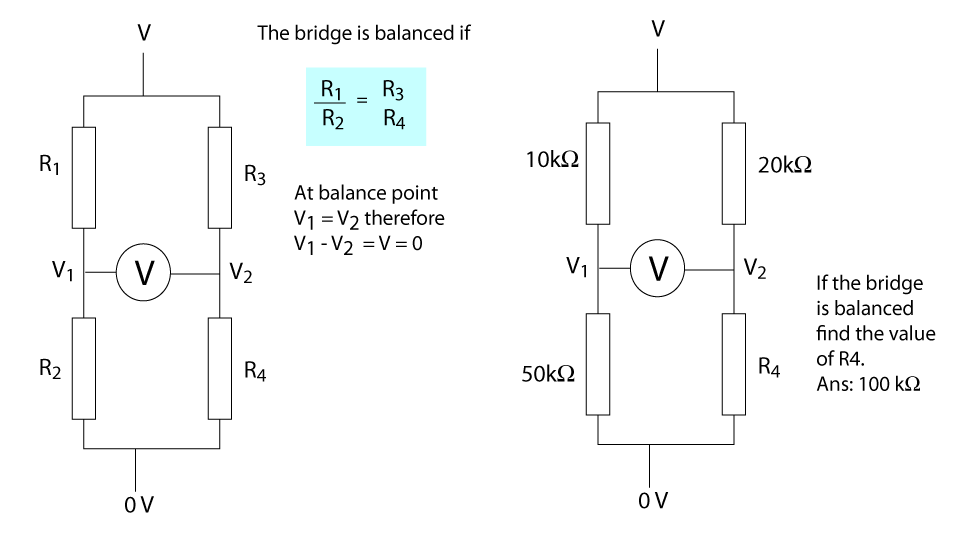## 15) Out of balance Wheatstone bridge

If we have a balanced Wheatstone bridge circuit and gradually change one of the resistors, R4 say, then the out of balance voltage is directly proportional to the out of balance resistance.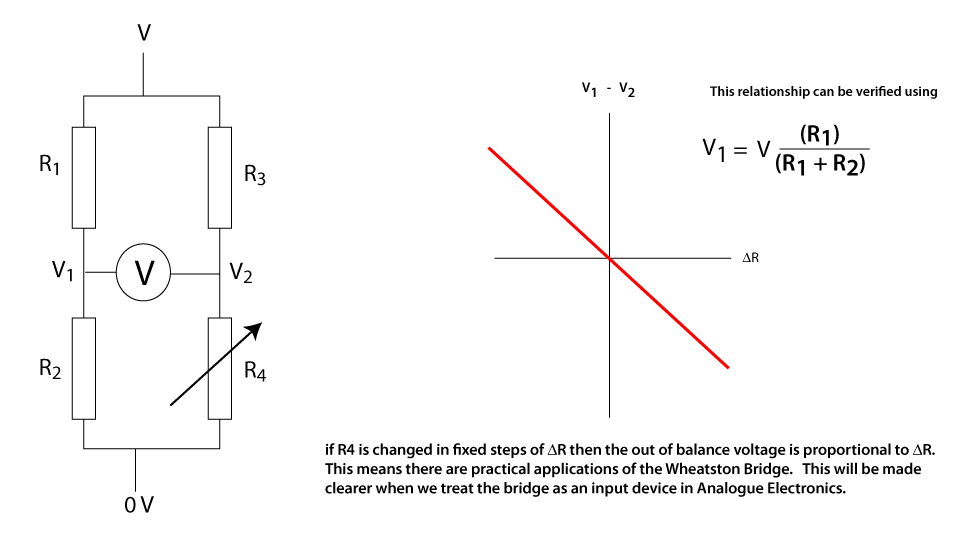## 17) Using circuit terms in context

Before we can use the following terms in context; charge, current, p.d., resistance, terminal p.d., load resistor, bridge circuit, e.m.f , lost volts, short circuit current, we should be able to explain each term in our own words using the definition or diagram where possible. Look at the circuit below and study closely how each term has been used.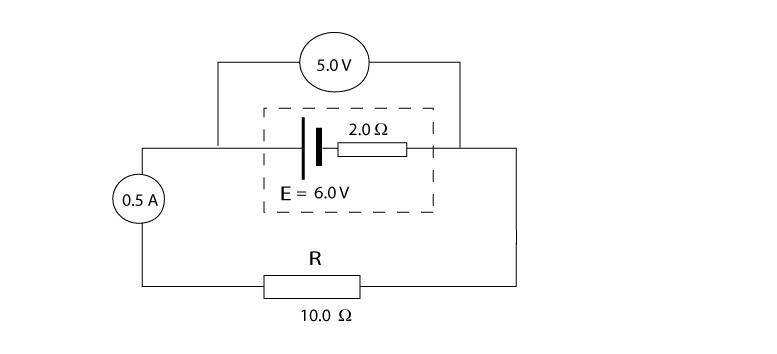The EMF of the cell is 6.0 V, this means 6 J of energy is supplied to each coulomb of charge that passes through the cell. The internal resistance, r = 2 W, is the resistance associated with the cell itself. This resistance is responsible for energy losses in the cell and thus is not available for the load resistor, R. This energy lost within the cell per coulomb is called the lost volts and in the example above is equal to 1.0 V. The current in the circuit is equal to 0.5 A. This means 0.5 coulombs of charge pass each point in the circuit per second, where one coulomb is equal to 6.0 x 1018 charges ie electrons. The terminal potential difference across the load resistor (and cell) is 5.0 V, this means 5.0 J of electrical energy per Coulomb is available to the resistor. The short circuit current is the total current the cell can supply and is found by replacing the load resistance by a wire of zero resistance. (or connecting a wire in parallel with the load resistor). In the example above the short circuit current is 3.0 A. You should verify that these numbers are consistent with respect to each other. Note: Resistance is explained on the left hand column. A bridge circuit is two voltage dividers in parallel with a voltmeter between them as in the Wheatstone Bridge.